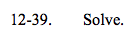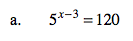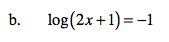### Home > A2C > Chapter 12 > Lesson 12.1.2 > Problem12-39

12-39.
1. Solve. Homework Help ✎

1. 5x−3 = 120

2. log(2x + 1) = −1Use the properties of logarithms.

log(5x − 3) = log(120)
(x − 3)(log5) = log120

$x-3=\frac{\text{log}120}{\text{log}5}$

$x=3+\frac{\text{log}120}{\text{log}5}\approx5.97$$-\frac{9}{20}$